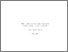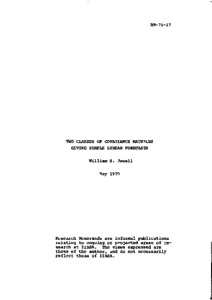Two Classes of Covariance Matrices Giving Simple Linear Forecasts

Jewell, W.S. (1975). Two Classes of Covariance Matrices Giving Simple Linear Forecasts. IIASA Research Memorandum. IIASA, Laxenburg, Austria: RM-75-017Preview
Text
RM-75-017.pdf

Abstract

Two special classes of covariance matrices are considered which give simplified computations for linear forecasts without continued reinversion of the matrix. In the first class, the optimal coefficients in the forecast can be computed in advance for every time period by simple closed formulas. In the second class, which is a generalization of the first, the optimal coefficients are obtained through a simple first-order linear recursive relation between forecasts of successive time periods. Collective risk forecasting models which give rise to these classes of covariances are presented.

Item Type: Monograph (IIASA Research Memorandum) System and Decision Sciences - Core (SDS) IIASA Import 15 Jan 2016 01:43 27 Aug 2021 17:08 http://pure.iiasa.ac.at/499View Item